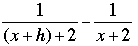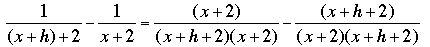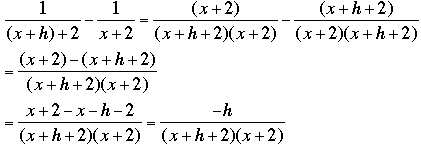SEARCH HOMEMath Central Quandaries & QueriesQuestion from Amanda, a student: It has been a really long time since I was in Algebra and I can't remember how to factor cubes such as x^3 +81 or subtracting/adding fractions with variables such as [1/(x+h)+2]-[1/x+2]. Please help!!!Hi Amanda,

The expressions for factoring the sum and difference of cubes are

x3 + y3 = (x + y)(x2 - xy + y2)
x3 - y3 = (x - y)(x2 + xy + y2)

If you add or subtract algebraic fractions you need to find a common denominator just as in adding or subtracting numerical fractions. For your examplea common denominator is (x + h + 2)(x + 2). Thus multiply the numerator and denominator of the first fraction by x + 2 and the numerator and denominator of the second fraction by x + h + 2. This givesNow that the fractions have the same denominator you can subtract the numeratorsI hope this helps,
PennyMath Central is supported by the University of Regina and The Pacific Institute for the Mathematical Sciences.